# How to Randomize List in Microsoft Excel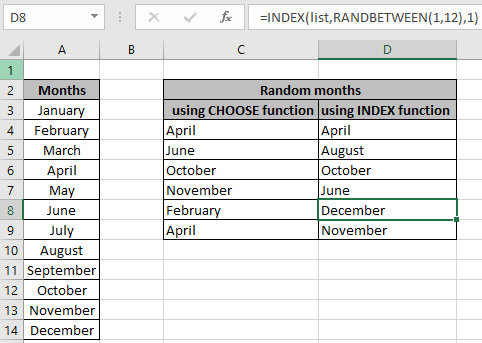In this article, we will learn How to generate random values from the given list or table column in excel.

Scenario :

For Instance, when working with values in a list or table in Excel. we need to find the random sample of values from the given list or table. For this generally we use the combination of CHOOSE and RANDBETWEEN function or it can be done by using the combination of INDEX function, ROWS function and RANDBETWEEN function. Let's make a formula out of these functions and try it on a sample data.

How to solve the problem?

For this, we can opt for any of the two methods mentioned below. One method is using CHOOSE function. In this We need to input all individual values from the list to the function. You can use this formula for when number values in the list are less.

Formula using CHOOSE function:

 = CHOOSE ( RANDBETWEEN ( 1 , n) , "value1" , "value2" , "value3",,,"value n" )

n : number of values in list

value1 , value2 , value3 , till value n : individual values from the list. These values can be input either using the cell reference or using directly with quotes or using the cell reference.

Another method is using the INDEX function where we will feed a random number as index which will result in the corresponding value at the index.

Formula using INDEX function:

 =INDEX(data, RANDBETWEEN (1,ROWS (data)),col_index)

data : data array can be a list or table.

1 : start index

col_index : column index to point out the list in table.

Example :

All of these might be confusing to understand. Let's understand these formulas using it in a list and table in excel. Here we have a list of months and we need some random months values from the months list.

Use the formula:

 = CHOOSE ( RANDBETWEEN ( 1, 12 ), \$A\$3,\$A\$4,\$A\$5,\$A\$6,\$A\$8,\$A\$7,\$A\$9,\$A\$10,\$A\$11,\$A\$12,\$A\$13,\$A\$14)

Explanation

1. RANDBETWEEN ( 1, 12 ) returns a random value from the 1 to 12.
2. Rest are the individual values from the array given using absolute cell reference.
3. CHOOSE function returns the value from the input list of values corresponding to the generated number.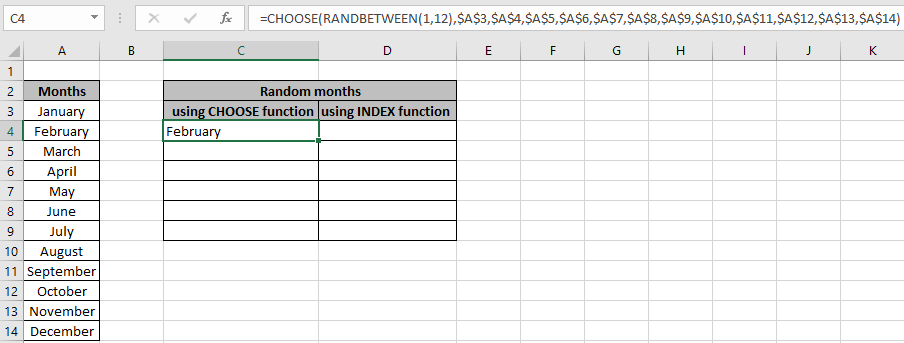The \$ sign with cell reference freezes the cell reference when formula is copied to the other cells. Copy and paste the formula for the number of required random sample using the Ctrl + D or dragging down from the bottom right corner of the selected cell.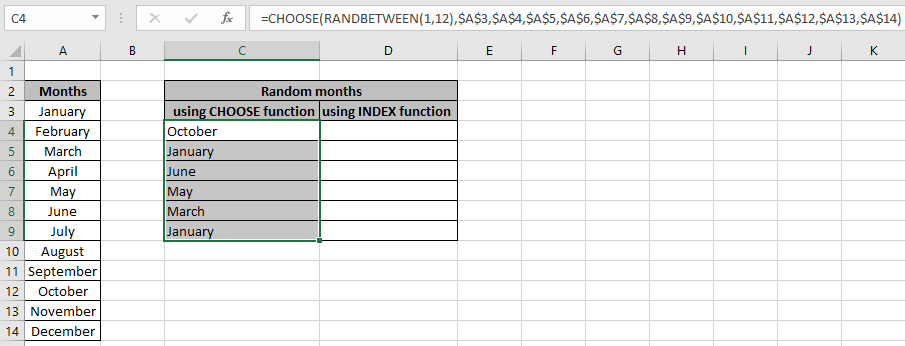As we can see the random results from the list using the CHOOSE formula in Excel. Now use the INDEX formula, this is more helpful than the CHOOSE formula as it doesn't require to input individual values. You can provide the fixed array using the naming the list using named range.

Use the formula :

 =INDEX ( table , RANDBETWEEN (1 , ROWS(table)), 1 )

list : named range used for A3:A14.

Explanation :

1. ROWS(table) returns the number of rows in the table which will be the last index of value in the list which is 12.
2. RANDBETWEEN function returns a random number from 1 to 12.
3. INDEX function will return the value corresponding to the returned number in the first column as list has only one column.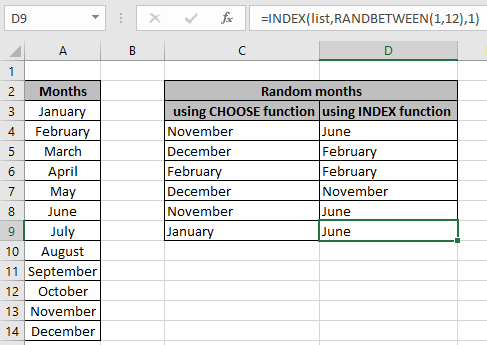This is much simpler and easier. Now we will use the above formula for the value in the table.

Generate random value from the table:

Here given a table and requiring random values from the particular column. We need to find the random item names from the first column of the table.

Use the formula:

 = CHOOSE ( RANDBETWEEN ( 1, 12 ), \$A\$3,\$A\$4,\$A\$5,\$A\$6,\$A\$8,\$A\$7,\$A\$9,\$A\$10,\$A\$11,\$A\$12,\$A\$13,\$A\$14)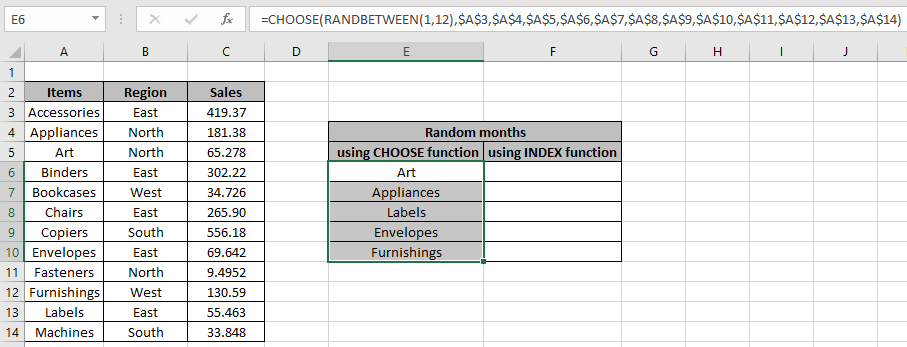Here we used the formula to extract different item names in Excel. Now use the other INDEX formula for the same.

Use the formula :

 =INDEX ( table , RANDBETWEEN (1 , ROWS(table)), 1 )

list : named range used for A3:A14.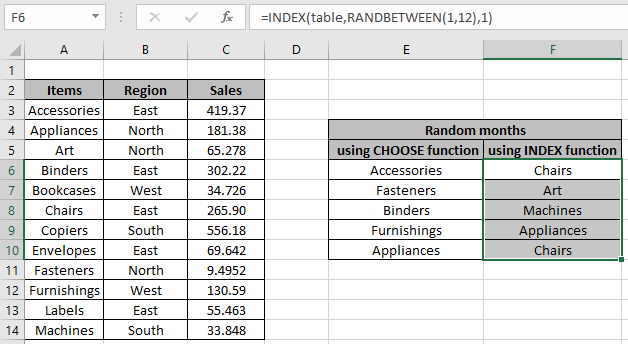Index function formula is much easier and can be applied over any column in the table just changing the col_index argument to the required column number.

RANDBETWEEN refreshes every time when something gets changed in the workbook. So when you are satisfied with the random data. Copy and paste values using the Paste special shortcut to get fixed values. You can learn how to get the random numbers or random date values in Excel

Here are all the observational notes regarding using the formula.
Notes :

If the returned number by the RANDBETWEEN function exceeds the number of values in the list, then the function returns error.

Hope this article about How to Randomize List in Microsoft Excel is explanatory. Find more articles on generating random numbers or date values here. If you liked our blogs, share it with your fristarts on Facebook. And also you can follow us on Twitter and Facebook. We would love to hear from you, do let us know how we can improve, complement or innovate our work and make it better for you. Write us at info@exceltip.com

Related Articles :

All About Excel Named Ranges : excel ranges that are tagged with names are easy to use in excel formulas. Learn all about it here.

Relative and Absolute Reference in Excel : Understanding of Relative and Absolute Reference in Excel is very important to work effectively on Excel. Relative and Absolute referencing of cells and ranges.

Generate Random Phone Numbers : Generate random 10 digti numbers using the RANDBETWEEN formula in Excel.

Get Random number From Fixed Options : Generate random numbers form the list having criteria in Excel.

Get Random numbers between two numbers : RANDBETWEEN function generator number between the two given numbers in Excel.

Excel Random Selection: How to Get Random Sample From a Dataset : Use the random samples in Excel for the explained examples here.

How to use the RANDBETWEEN Function in Excel : Excel RANDBETWEEN function returns a number between two given numbers in Excel.

Popular Articles :

How to use the IF Function in Excel : The IF statement in Excel checks the condition and returns a specific value if the condition is TRUE or returns another specific value if FALSE.

How to use the VLOOKUP Function in Excel : This is one of the most used and popular functions of excel that is used to lookup value from different ranges and sheets.

How to Use SUMIF Function in Excel : This is another dashboard essential function. This helps you sum up values on specific conditions.

How to use the COUNTIF Function in Excel : Count values with conditions using this amazing function. You don't need to filter our data to count specific values. Countif function is essential to prepare your dashboard.

Terms and Conditions of use

The applications/code on this site are distributed as is and without warranties or liability. In no event shall the owner of the copyrights, or the authors of the applications/code be liable for any loss of profit, any problems or any damage resulting from the use or evaluation of the applications/code.